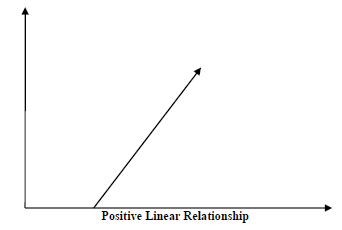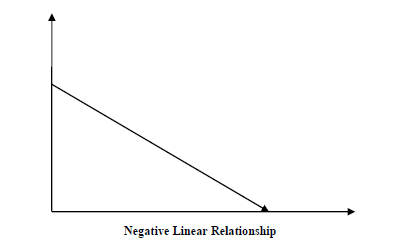# Regression Algorithms - Linear Regression

## Introduction to Linear Regression

Linear regression may be defined as the statistical model that analyzes the linear relationship between a dependent variable with given set of independent variables. Linear relationship between variables means that when the value of one or more independent variables will change (increase or decrease), the value of dependent variable will also change accordingly (increase or decrease).

Mathematically the relationship can be represented with the help of following equation −

$$Y\:=\:mX+b$$

Here, Y is the dependent variable we are trying to predict.

X is the independent variable we are using to make predictions.

m is the slop of the regression line which represents the effect X has on Y

b is a constant, known as the 𝑌Y-intercept. If X = 0,Y would be equal to 𝑏b.

Furthermore, the linear relationship can be positive or negative in nature as explained below −

### Positive Linear Relationship

A linear relationship will be called positive if both independent and dependent variable increases. It can be understood with the help of following graph −### Negative Linear relationship

A linear relationship will be called positive if independent increases and dependent variable decreases. It can be understood with the help of following graph −## Types of Linear Regression

Linear regression is of the following two types −

## Assumptions

The following are some assumptions about dataset that is made by Linear Regression model −

Multi-collinearity − Linear regression model assumes that there is very little or no multi-collinearity in the data. Basically, multi-collinearity occurs when the independent variables or features have dependency in them.

Auto-correlation − Another assumption Linear regression model assumes is that there is very little or no auto-correlation in the data. Basically, auto-correlation occurs when there is dependency between residual errors.

Relationship between variables − Linear regression model assumes that the relationship between response and feature variables must be linear.